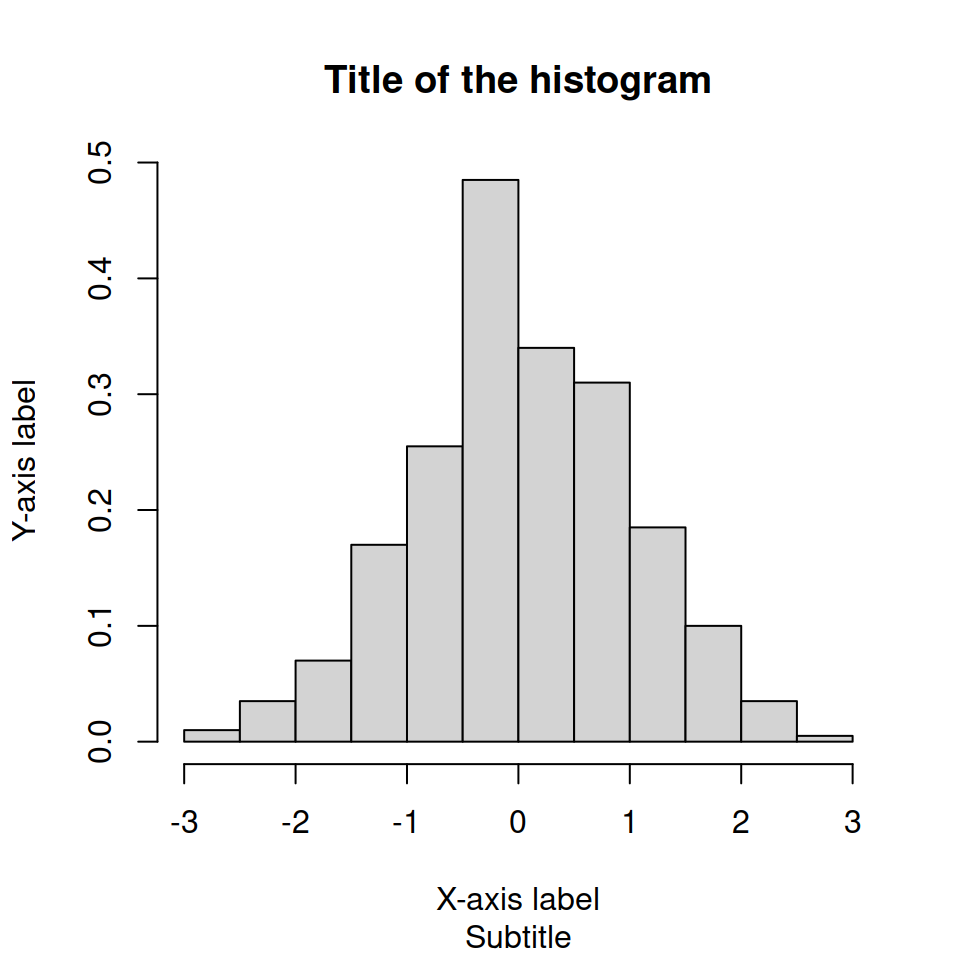# Density histogram in R

## A basic density histogram

The `hist` function creates frequency histograms by default. You can override this behaviour by setting `prob = TRUE` or `freq = FALSE`.

``````# Sample data (normal)
set.seed(1)
x <- rnorm(400)

# Histogram
hist(x, prob = TRUE)
hist(x, freq = FALSE) # Equivalent``````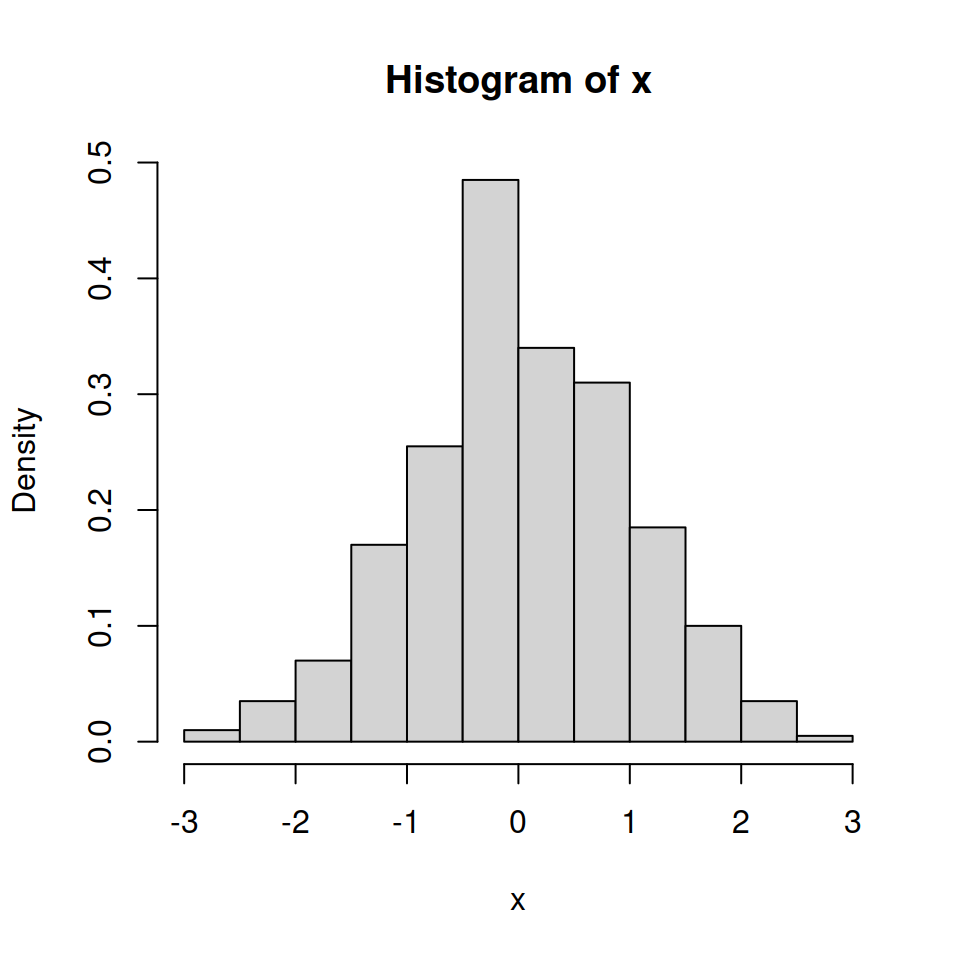## Color of the histogram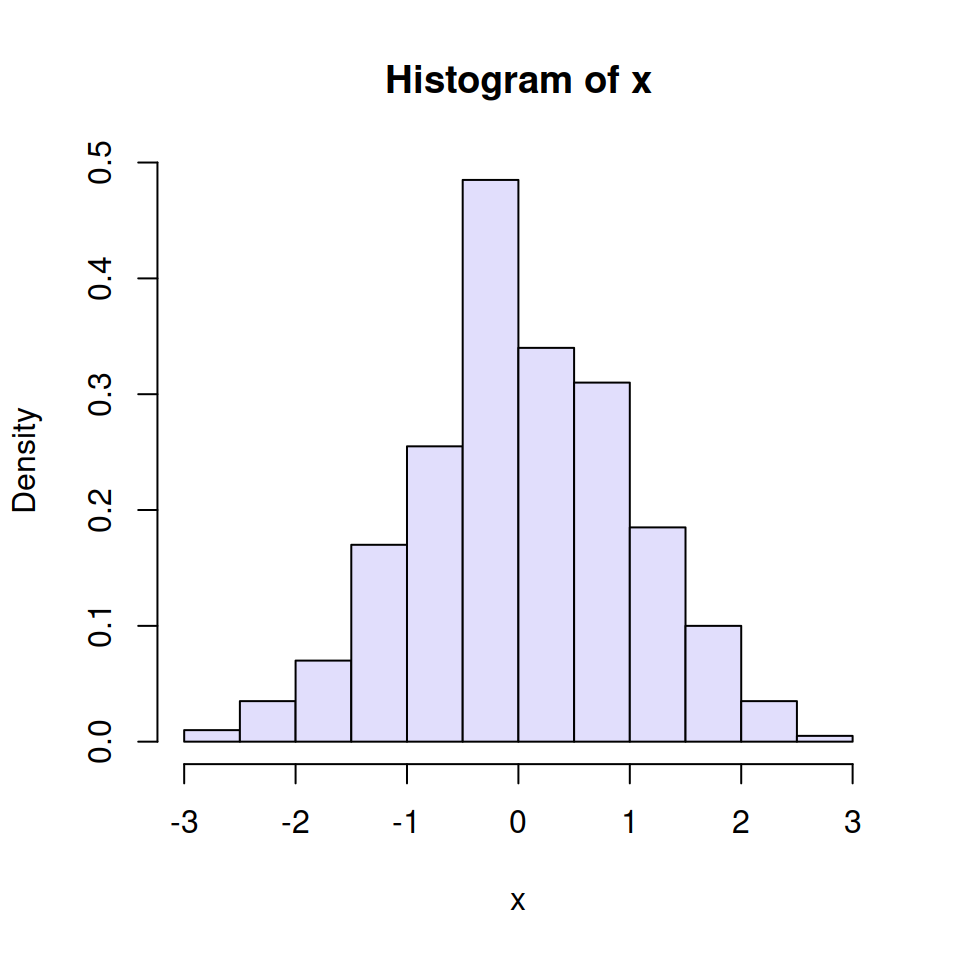You can change the default fill color of the histogram bars (gray since R 4.0.0) to other making use of the `col` argument.

``````# Sample data (normal)
set.seed(1)
x <- rnorm(400)

# Blue histogram
hist(x,
prob = TRUE,
col = "#E1DEFC") # Color``````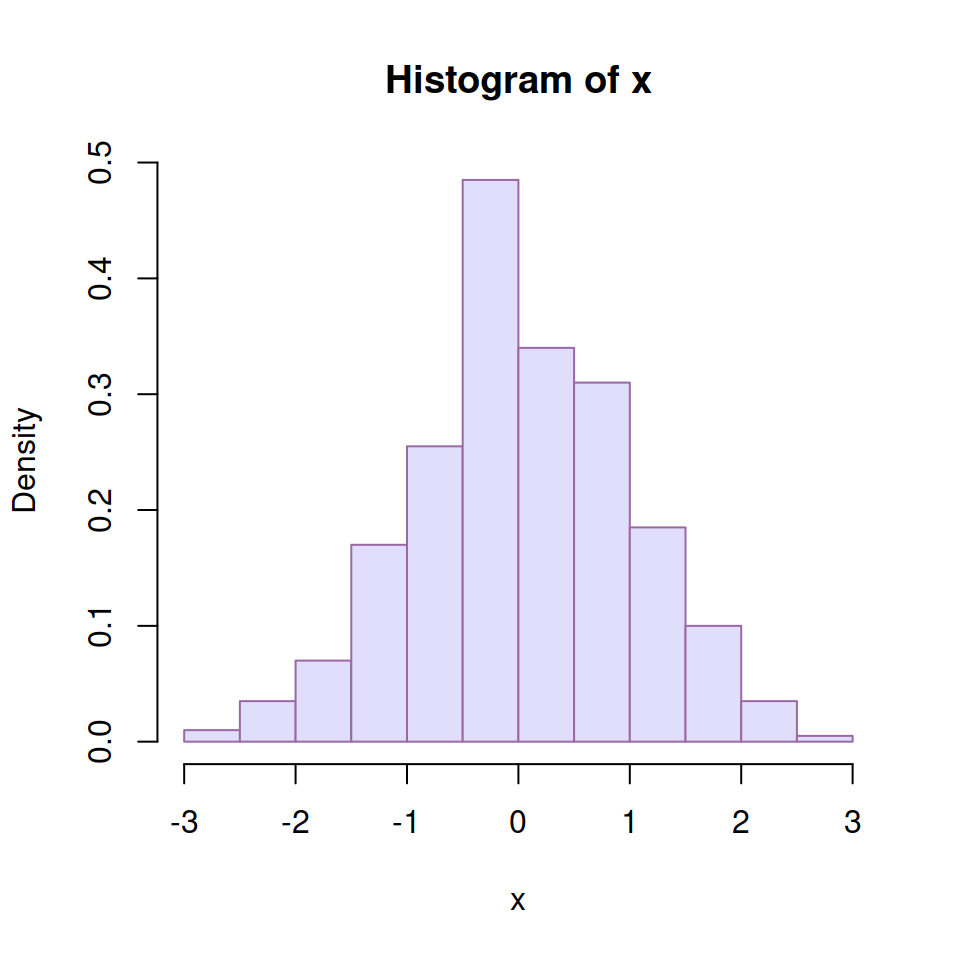The `border` argument allows modifying the border color of the bars.

``````# Sample data (normal)
set.seed(1)
x <- rnorm(400)

# Purple histogram
hist(x,
prob = TRUE,
col = "#E1DEFC",    # Fill color
border = "#9A68A4") # Border``````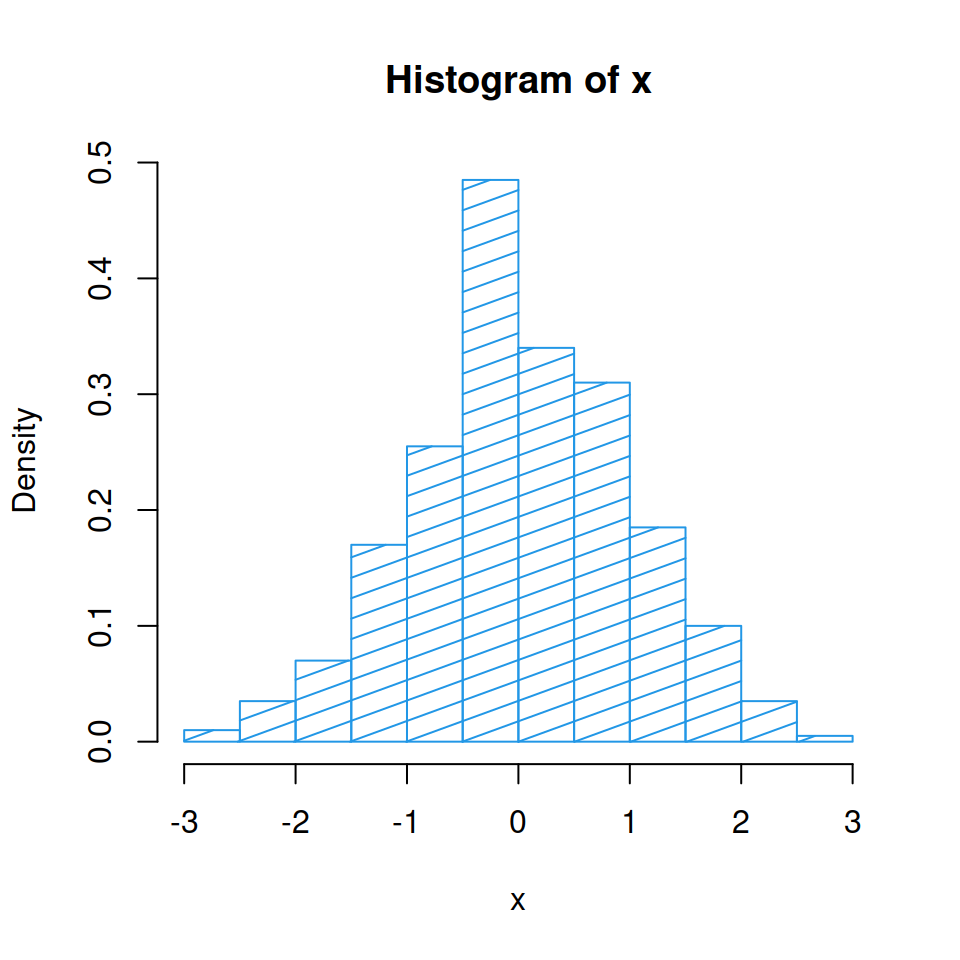You can also use shading lines instead of a fill color. Set them with the `density` argument and modify its angle with `angle`.

``````# Sample data (normal)
set.seed(1)
x <- rnorm(400)

# White histogram with shading lines
hist(x,
prob = TRUE,
col = 4,      # Color
density = 10, # Shading lines
angle = 20)   # Shading lines angle``````

## Titles and labels

You can also modify the title, subtitle, and axes labels with `main`, `sub`, `xlab` and `ylab` arguments, respectively.

``````# Sample data (normal)
set.seed(1)
x <- rnorm(400)

# Histogram with titles
hist(x,
prob = TRUE,
main = "Title of the histogram", # Title
sub = "Subtitle",                # Subtitle
xlab = "X-axis label",           # X-axis label
ylab = "Y-axis label")           # Y-axis label``````